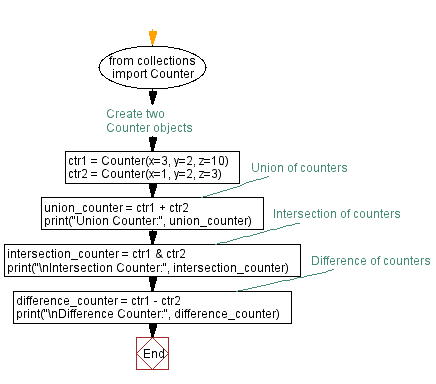﻿ Python program for Counter operations: Union, intersection, and difference

# Python Program: Counter operations - Union, intersection, and difference

## Python Counter Data Type: Exercise-4 with Solution

Write a Python program that creates two 'Counter' objects and finds their union, intersection, and difference.

Sample Solution:

Code:

``````from collections import Counter

# Create two Counter objects
ctr1 = Counter(x=3, y=2, z=10)
ctr2 = Counter(x=1, y=2, z=3)

# Union of counters
union_counter = ctr1 + ctr2
print("Union Counter:", union_counter)

# Intersection of counters
intersection_counter = ctr1 & ctr2
print("\nIntersection Counter:", intersection_counter)

# Difference of counters
difference_counter = ctr1 - ctr2
print("\nDifference Counter:", difference_counter)
``````

Output:

```Union Counter: Counter({'z': 13, 'x': 4, 'y': 4})
Intersection Counter: Counter({'z': 3, 'y': 2, 'x': 1})
Difference Counter: Counter({'z': 7, 'x': 2})
```

In the exercise above, two "Counter" objects 'ctr1' and 'ctr2' are created with different key-value pairs. The union operation '+' adds up the counts of the common keys in both counters. The intersection operation '&' keeps the minimum count of common keys. The difference operation '-' subtracts the count of keys in the second counter from the first counter. Finally each case's results are printed.

Flowchart:What is the difficulty level of this exercise?

Test your Programming skills with w3resource's quiz.

﻿

## Python: Tips of the Day

Summing a sequence of numbers (calculating the sum of zero to ten with skips):

```>>> l = range(0,10,2)
>>> sum(l)
20
```

We are closing our Disqus commenting system for some maintenanace issues. You may write to us at reach[at]yahoo[dot]com or visit us at Facebook